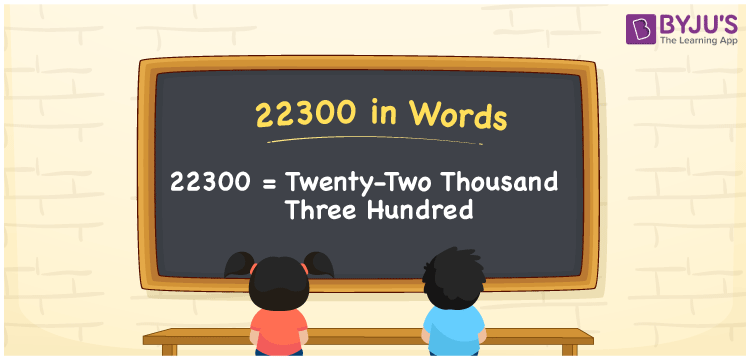# 22300 in Words

22300 in words can be written as Twenty-Two Thousand Three Hundred. If you buy a treadmill for Rs. 22300, then you can say that “I bought a treadmill for Twenty-Two Thousand Three Hundred Rupees”. For details of the numbers in words concept, refer to the various study materials designed by the subject experts. Hence, 22300 can be read as “Twenty-Two Thousand Three Hundred” in English.

 22300 in words Twenty-Two Thousand Three Hundred Twenty-Two Thousand Three Hundred in Numbers 22300

## 22300 in English Words## How to Write 22300 in Words?

There are five digits in 22300 and the place value of each digit can be found in the table given below.

 Ten Thousands Thousands Hundreds Tens Ones 2 2 3 0 0

The expanded form of 22300 is:

2 x Ten Thousand + 2 x Thousand + 3 × Hundred + 0 × Ten + 0 × One

= 2 x 10000 + 2 x 1000 + 3 x 100

= 20000 + 2000 + 300

= 22300

= Twenty-Two Thousand Three Hundred

Therefore, 22300 in words is written as Twenty-Two Thousand Three Hundred.

22300 is a natural number that precedes 22301 and succeeds 22299.

22300 in words – Twenty-Two Thousand Three Hundred

Is 22300 an odd number? – No

Is 22300 an even number? – Yes

Is 22300 a perfect square number? – No

Is 22300 a perfect cube number? – No

Is 22300 a prime number? – No

Is 22300 a composite number? – Yes

## Frequently Asked Questions on 22300 in Words

Q1

### Write 22300 in words.

22300 can be written in words as “Twenty-Two Thousand Three Hundred”.
Q2

### How do you write Twenty-Two Thousand Three Hundred in numbers?

Twenty-Two Thousand Three Hundred can be written in numbers as 22300.
Q3

### Is 22300 a perfect cube number?

No, 22300 is not a perfect cube number as it is not the product of three similar numbers.